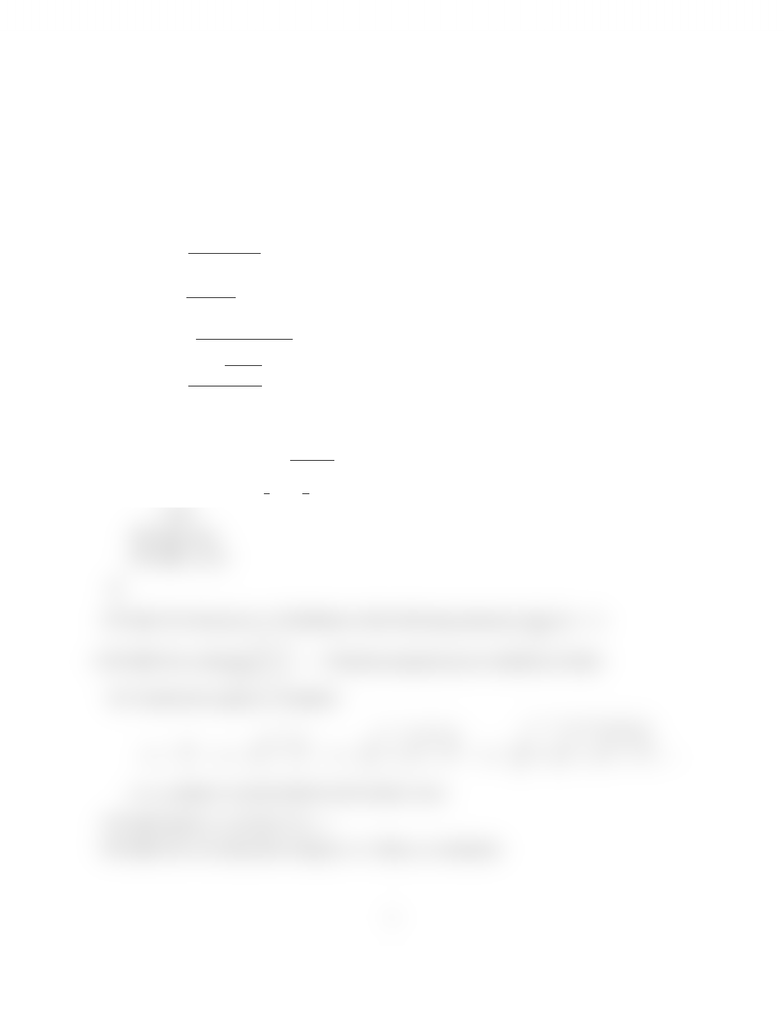Study Guides (390,000)
CA (150,000)
UTSG (10,000)
MAT (1,000)
MAT137Y1 (100)
N/ A (30)
Midterm

# TEST 1

Department
Mathematics
Course Code
MAT137Y1
Professor
N/ A
Study Guide
Midterm

This preview shows half of the first page. to view the full 2 pages of the document.Department of Mathematics, University of Toronto
Term Test 1–November 15, 2006
MAT137Y,Calculus!
Time Alloted: 1hour 50 minutes
1. Evaluate the following limits. Do not use L’H ˆ
opital’sRule to evaluate the limit.
(7%) (i) lim
x0
(x1)21
x2+6x
.
(7%) (ii) lim
t0
sin2(5t)
3t2.
(7%) (iii) lim
x4+
(4x)|3x14|
|4x|
.
(7%) (iii) lim
x0
3
9x2
x2.
2.
(7%) (i) Solvethe inequality
x23x
x41
(ii) Suppose sin x=3
4and π
2xπ.Find the exact value of each of the following expres-
sions.
(6%) (a) tan x.
(4%) (b) cos 2x.
3.
(5%) (a) Givethe precise ε,δdeﬁnition of the following statement: lim
xa
f(x)=L.
(12%) (b) Provethat lim
x3
x2+1
1x
=5directly using the precise deﬁnition of limit.
4. Consider the sequence of numbers
x1=
1,x2=
q
1+
1,x3=
r
1+
q
1+
1,x4=
s
1+
r
1+
q
1+
1,...,
so xncontaines nnested radicals and exactly nones.
(3%) (a) Express xnin terms of xn1.
(9%) (b) Provefor all positiveintegers n2that xnis irrational.
1
www.notesolution.com